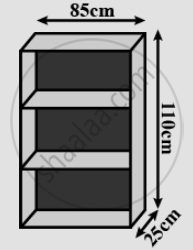# A Wooden Bookshelf Has External Dimensions as Follows: Height = 110 Cm, Depth = 25 Cm, Breadth = 85 Cm (See Fig. 18.5). - Mathematics

A wooden bookshelf has external dimensions as follows: Height = 110 cm, Depth = 25 cm, Breadth = 85 cm in following figure. The thickness of the plank is 5 cm everywhere. The external faces are to be polished and the inner faces are to be painted. If the rate of polishing is 20 paise per cm2 and the rate of painting is 10 paise per cm2. Find the total expenses required for polishing and painting the surface of the bookshelf.#### Solution

External length of book shelf = 85cm = l
Height = 110cm.
External surface area of shelf while leaving front face of shelf

=lh+2(lb+bh)

=[85xx110+21(85xx25+25xx110)]"cm"^2

= 19100"cm"^2

Area of front face = (85xx110-75xx100+2(75xx5))"cm"^2

=1850+ 750"cm"^2

=2600"cm"^2

Area to be polished = 19100+2600"cm"^2

=21700"cm"^2

Cost of polishing 1"cm"^2"area" = "Rs". 0.20

Cost of polishing 21700"cm"^2 are = "Rs".[21700xx0.20]
= "Rs". 4340

Now, length (l), breath (b), height (h) of each row of book shelf is 75cm, 20cm and 30cm

((110-20)/3) respectively.

Area to be painted in row = 2(l+h)b+lh

=[2(75+30)xx20+75xx30]"cm"^2

=(4200+2250)"cm"^2

=6450"cm"^2

Area to be painted in 3 rows =(3xx6450)"cm"^2

=19350"cm"^2
"Cost of painting"  1"cm"^2 area = Rs. 0.10

"Cost of painting 19350 area" = Rs.(19350 xx 0 .10) - "Rs".1935

Total expense required for polishing and painting the surface of the bookshelf

= Rs.(4340 +1935) = Rs. 6275.

Concept: Surface Area of a Cuboid
Is there an error in this question or solution?

#### APPEARS IN

RD Sharma Mathematics for Class 9
Chapter 18 Surface Areas and Volume of a Cuboid and Cube
Exercise 18.1 | Q 19 | Page 15

Share